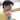# Writing a sentence without using the Alphabet

July 20, 2019

Read the originalImprove this translationView all translated posts

`(+!![] / +![] + [])[!+[] + !+[] + !+[]] +   (+!![] / +![] + [])[+!+[]] +   (![] + {})[+!+[] + [+[]]] +   (!![] + [])[+!+[]] +   (!![] + [])[!+[] + !+[] + !+[]] +   ([][[]] + [])[!+[] + !+[]] +   (+!![] / +![] + [])[!+[] + !+[] + !+[]] +   ([] + {})[!+[] + !+[]] +   (![] + [])[!+[] + !+[]] +   (!![] + [])[!+[] + !+[] + !+[]]// -> 'incredible'`

It’s hard to believe, but when you run the above code on the browser’s developer console, you’ll see `incredible`. This is not a bug, and it’s normal code that uses tricky JavaScript features.

That weird code displays the message using JavaScript’s type conversion in addition.

## Weird JavaScript generator

Enter the message to be converted in the input form below. Then copy the generated weird code to the clipboard and run it in the browser’s developer console or node.js REPL environment to view the original message.

`aladdin` · `babel` · `bts` · `california` · `fail` · `incredible` · `nodejs` · `octocat` · `r2d2` · `san francisco` · `seoul` · `starcourt` · `success` · `sydney` · `to infinity and beyond` · `toy story 3` · `yoda`
`(![] + [])[!+[] + !![] + !![]] +   // 's' == 'false'  (!![] + [])[+[]] +               // 't' == 'true'  (![] + [])[+!![]] +              // 'a' == 'false'  (!![] + [])[+!![]] +             // 'r' == 'true'  (![] + {})[+!![] + [+[]]] +      // 'c' == ('false' + '[object Object]')  ([] + {})[+!![]] +               // 'o' == '[object Object]'  ([][[]] + [])[+[]] +             // 'u' == 'undefined'  (!![] + [])[+!![]] +             // 'r' == 'true'  (!![] + [])[+[]]                 // 't' == 'true'// -> 'starcourt'`

Only numbers and alphabets are converted, but the following alphabets are not converted.

`g` `h` `k` `m` `p` `q` `v` `w` `x` `z`

If you know how to convert the those alphabets, please let me know. thank you.

## Brief explanation

A similar pattern is shown on each line of the result code.

`([]+{})[!+[]+!+[]] == ( []+{} )[ !+[]+!+[] ]  // 'b'([]+{})[+!+[]]     == ( []+{} )[ +!+[] ]      // 'o'(+{}+[])[+!+[]]    == ( +{}+[] )[ +!+[] ]     // 'a'(!![]+[])[+[]]     == ( !![]+[] )[ +[] ]      // 't'// ( string )[ n ]`

You can get the character of the nth index from any string in the same way as above, and the strings and numbers used in the conversion can be obtained by the following ways.

`+![]            // 0+!![]           // 1!![]            // true![]             // false[][[]]          // undefined+!![] / +![]    // Infinity[] + {}         // "[object Object]"+{}             // NaN`

This post was deeply inspired by What the f*ck JavaScript?.

The conversion principle is described in detail in the article above. and it is easier to understand by referring to the explanation comment of the generator results.

Edit on GitHubI am a developer dad with a son who dreams of a developer.
I'm interested in data visualization and enjoy creating fun programs.